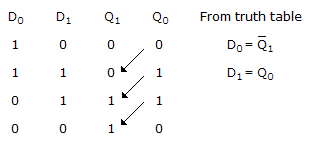# Electronics and Communication Engineering - Exam Questions Papers

6.

The transfer function Y(s)/R(s) of the system shown is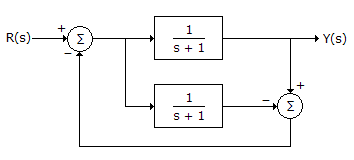A. 0 B.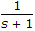C.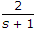D.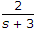Explanation:

Construct a signal flow graph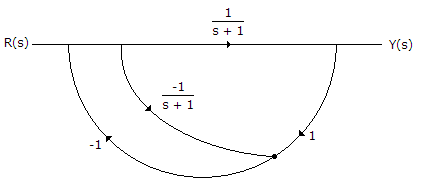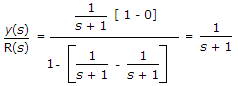.

7.

A lossless transmission line with air dielectric is 6 m long. What is the line length at 30 MHz?

 A. 1 wavelength long B. 0.5 wavelength long C. 0.6 wavelength long D. 0.25 wavelength long

Explanation:

V = 3 x 108 m/s for lossless line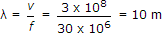Line length =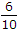= 0.6 wave length long.

8.

Given that F(s) is the one-sided Laplace transform of f(t), the Laplace transform of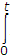f(t)dt is

 A. sFs - f(0) B.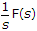C.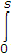F(t)dt D.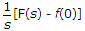Explanation:

Since it is one-sided Laplace transform.

9.

The antenna current of an AM broad cast transmitter, modulated to a depth of 40% by an audio sine wave is 11 amperes. It increased to 12 ampere as a result of simultaneous modulation by another sine wave. The modulation index due to this second sine wave is :

 A. 0.4 B. 0.757 C. 0.643 D. 0.8

Explanation: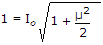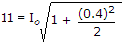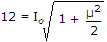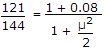μ2 = 0.57057, μ12 + 0.16 = 0.57057

⇒ μ1 = 0.64 .

10.

Two D flip-flop as shown below are to be connected as a synchronous counter that goes through the following Q1Q0 sequence
00 → 01 → 11 → 10 → 00 → __________
The inputs D0 and D1 respectively should be connected as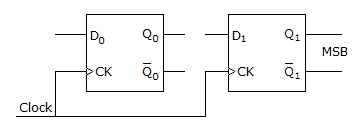A. Q1 and Q0 B. Q0 and Q1 C. Q1 Q0 and Q1 Q0 D. Q1 Q0 and Q1 Q0